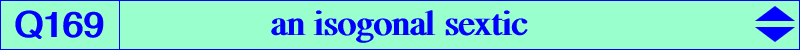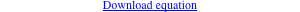∑ b^2 c^2 x^3 (y+z) [c^2 y (x-y+z) - b^2 z(x+y-z)] = 0X(1), X(3), X(4) excenters midpoints of ABC anti-points, see Table 77 infinite points of the 6 bisectors vertices of the two circum-perp triangles centers of the Apollonius circles points of K020 on the Lemoine axis their isogonal conjugates on the Steiner ellipse (S)Let P be a point in the plane of a triangle ABC, such that P is not situated on any of its sidelines, nor on its bisectors, nor on the circumcircle, nor on the line of infinity. Let La, Lab, Lac be the radical axes of the circle with center P passing through A, relatively to the circles (PBC), (PCA), (PAB). Let Ab = La ∩ Lab, Ac = La ∩ Lac and A' = BAb ∩ CAc. Define B' and C' cyclically. The lines AA', BB', CC' are concurrent (actually parallel) if and only if P is on the cubic K024 (Angel Montesdeoca, 2021-09-08). More generally, these lines AA', BB', CC' bound a triangle perspective (at Q) to ABC if and only if P lies on K024 (as above with Q at infinity) or P lies on Q169. *** Properties of Q169 A, B, C are nodes with tangents the symmedians and the sidelines of the antimedial triangle. The tangents at the in/excenters also pass through X(6). The tangents at O and H pass through X(66). Q169 has six real asymptotes parallel to the bisectors at the midpoint of the corresponding median. The isogonal conjugates of their infinite points are the vertices of the two circum-perp triangles A1B1C1 and A2B2C2, obviously on the circumcircle of ABC and on these bisectors. Q169 meets the Lemoine axis (trilinear polar of the Lemoine point) at the centers Ωa, Ωb, Ωc of the Apollonius circles and three other points which lie on K020. The isogonal conjugates of these latter three points lie on Q169, the Steiner ellipse and K020. Q169 meets K024 at A, B, C (each counting for three), Ωa, Ωb, Ωc and three pairs of Cayley's anti-points, on the perpendicular bisectors, on K003, see Table 77. *** A locus property (Angel Montesdeoca, 2022-01-23) PaPbPc denotes the pedal triangle of a point P, with respect to a triangle ABC. The perpendicular through P to PbPc intersects BC at A'. Similarly, points B' and C' are defined on CA and AB respectively. The lines AA', BB', CC' are concurrent (at Q) if and only if P lies on the sextic Q169. The perspector of triangles ABC and A'B'C' lies on a 12th degree curve.